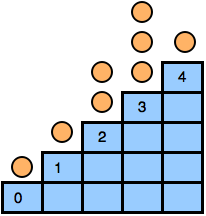# Intro to Staircase Nim

Let’s learn about Staircase Nim by solving move the coins problem that appeared in HackerRank April World Codesprint. Among the problems I have ever created, its one of my favorites. If you don’t want to solve that problem but want to learn about staircase-nim, just read the first part of this blog.

Staircase Nim

This problem is a variation of Staircase Nim problem, which is a not-very-well-known variation of classic Nim problem. If you don’t know what Nim Game is, I suggest you first to learn about it.

In Staircase nim, there is a staircase with n steps, indexed from $0$ to $n-1$. In each step, there are zero or more coins. See the figure below:Two players play in turns. In his/her move a player can choose a step $i>0$ and move one or more coins to step $i-1$. The player who is unable to make a move lose the game. That means the game ends when all the coins are in step $0$.

Now you have to decide who will win the game if both players play optimally.

Observation

We can divide the steps into two types, odd steps, and even steps. Now lets think what will happen if a player A move a coin from an even step to an odd step. Player B can move those coins to an odd position, and the state of the game won’t change.

But if A move a coin from an odd step to an even step, similar logic won’t work. Because there can be a situation where player B won’t be able to move those coins to another odd step to restore the state.

From this we can agree that coins in even steps are useless, they don’t affect game state. If I am in a winning position, and you move a coin from an even step, I will move those coins again to another even step and will remain in a winning position.

Determine Winning Position

Now we agreed that only coins to odd steps count. If you take one or more coins from an odd step and move them to an even step, the coins become useless! Remember even steps are useless, So moving to even step is just like throwing them away. Now we can imagine coins in an odd-step as a pile of stones in a standard nim game.Now it’s easy, just find the xorsum of all odd steps, and we are done!

Move the Coins

In the HackerRank problem, there is a tree and each node can contain zero or more coins.In each move, a player can move one or more coin from a node (which is not the root) to its parent node. If a player can’t make a move, he/she loses the game.

This is same as Staircase Nim but on a tree. You just need to calculate the distance between each node and the root. Now find the xorsum of all odd distance nodes. This can be done using a dfs, complexity $O(V+E)$.

Now, let’s discuss updating the xorsum for each question. Suppose $d_1$ is the distance between $1$ and $u$. For node $u$, you must *remove* the edge between $u$ and $p(u)$ and *add* an edge between $u$ and $v$. Suppose $d_2$ is the new distance between $u$ and $1$; if $d_1\%2 = d_2\%2$, it won’t change the xorsum because *odd* distance nodes still have *odd* distances (the same is true for *even* distance nodes).

If $d_1\%2 != d_2\%2$, all the *odd* distance nodes in subtree $u$ will become *even* distance nodes, and all the *even* distance nodes in subtree $u$ will become *odd* distance nodes. If you save the xorsum of *odd* and *even* distance nodes separately, you can easily update it for each question.

A question will be invalid if $u$ and $v$ belong to the same subtree. You can then easily find that using the finishing time in depth-first-search. Some did it by finding LCA but that’s too much.

The complexity of answering each question is $O(1)$.

That’s all! I will be grateful if you point out the mistakes.

[Originally published in Codeforces blog]